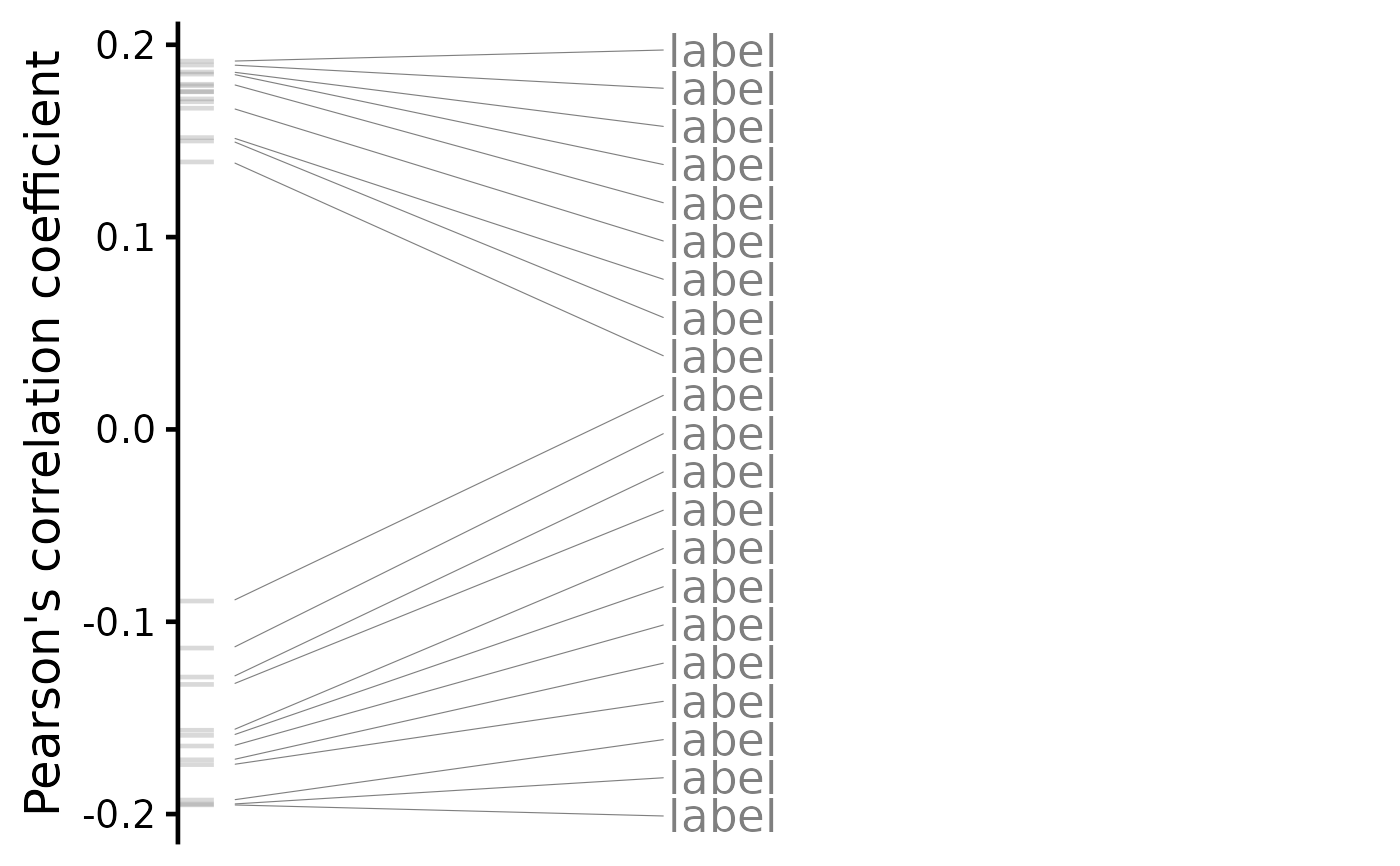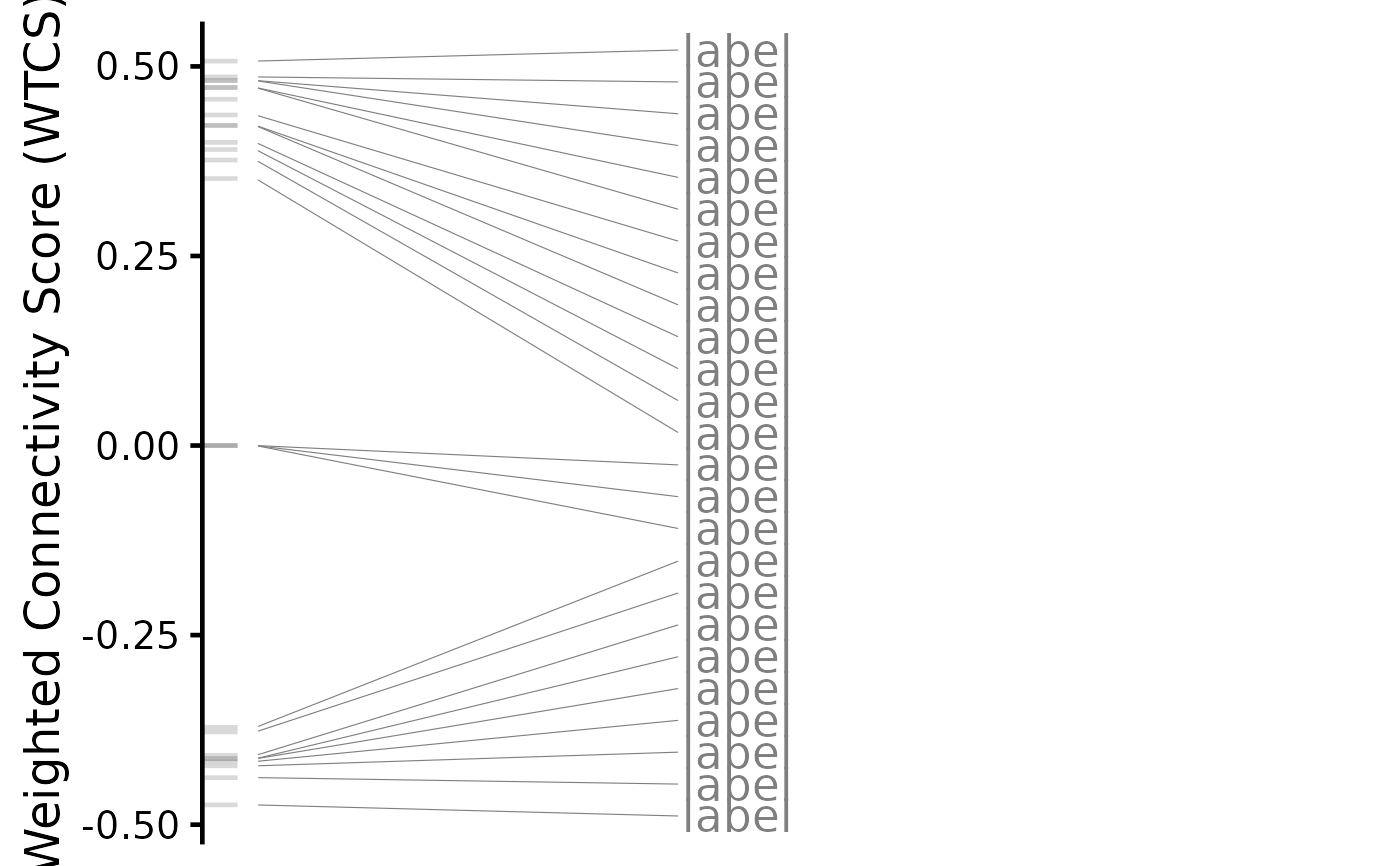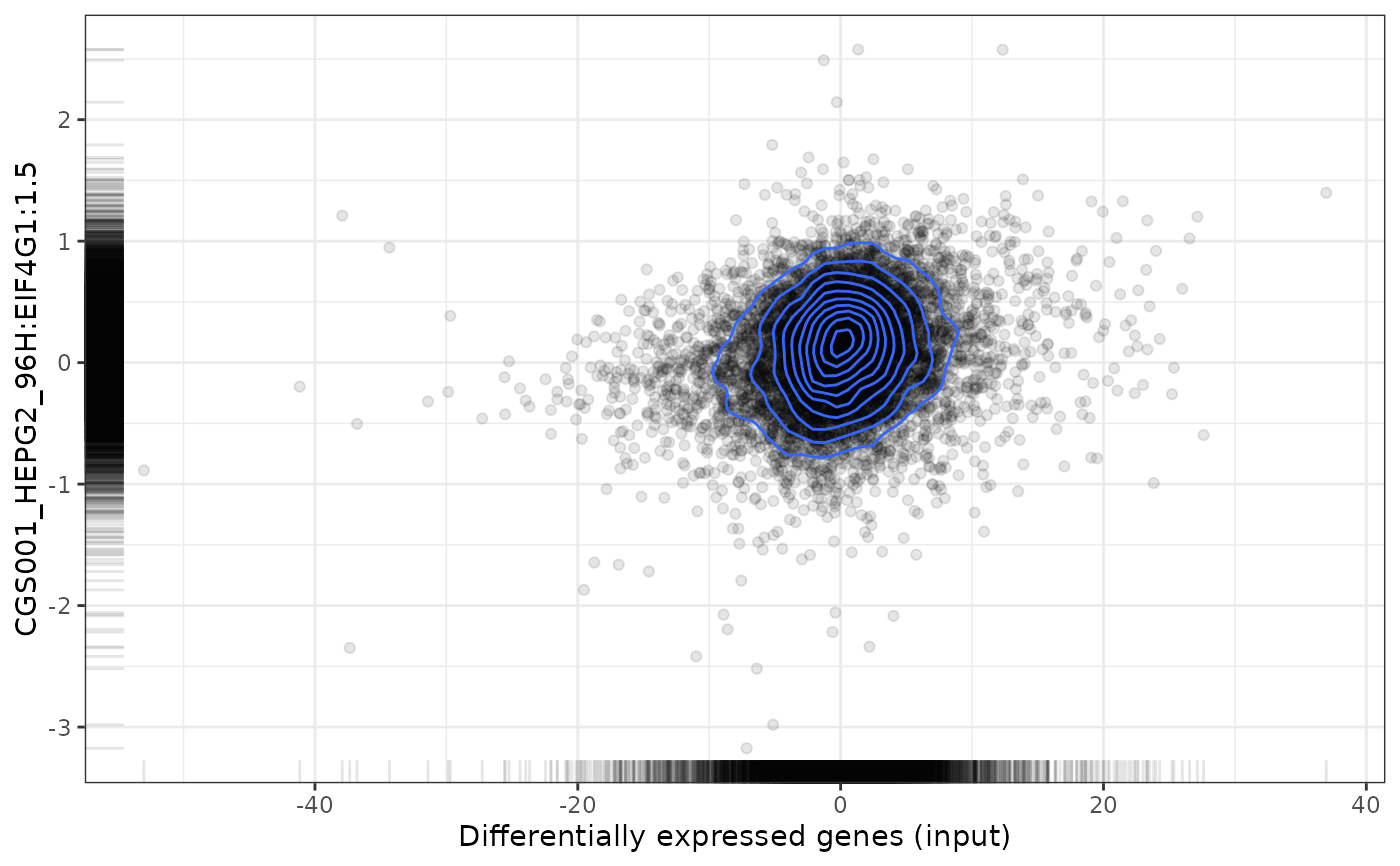If element = NULL, comparison is plotted based on all elements. Otherwise, show scatter or GSEA plots for a single element compared with previously given differential expression results.

# S3 method for referenceComparison
plot(
x,
element = NULL,
method = c("spearman", "pearson", "gsea", "rankProduct"),
n = c(3, 3),
plotNonRankedPerturbations = FALSE,
alpha = 0.3,
genes = c("both", "top", "bottom"),
...,
zscores = NULL,
title = NULL
)

## Arguments

x

referenceComparison object: obtained after running rankSimilarPerturbations() or predictTargetingDrugs()

element

Character: identifier in the first column of x

method

Character: method to plot results; choose between spearman, pearson, gsea or rankProduct (the last one is only available if element = NULL)

n

Numeric: number of top and bottom genes to label (if a vector of two numbers is given, the first and second numbers will be used as the number of top and bottom genes to label, respectively); only used if element = NULL

Boolean: show available metadata information instead of identifiers (if available)? Only used if element = NULL

plotNonRankedPerturbations

Boolean: plot non-ranked data in grey? Only used if element = NULL

alpha

Numeric: transparency; only used if element = NULL

genes

Character: when plotting gene set enrichment analysis (GSEA), plot most up-regulated genes (genes = "top"), most down-regulated genes (genes = "bottom") or both (genes = "both"); only used if method = "gsea" and geneset = NULL

...

Extra arguments currently not used

zscores

Data frame (GCTX z-scores) or character (respective filepath to load data from file)

title

Character: plot title (if NULL, the default title depends on the context; ignored when plotting multiple perturbations)

## Value

Plot illustrating the reference comparison

Other functions related with the ranking of CMap perturbations: as.table.referenceComparison(), filterCMapMetadata(), getCMapConditions(), getCMapPerturbationTypes(), loadCMapData(), loadCMapZscores(), parseCMapID(), plot.perturbationChanges(), plotTargetingDrugsVSsimilarPerturbations(), prepareCMapPerturbations(), print.similarPerturbations(), rankSimilarPerturbations()

Other functions related with the prediction of targeting drugs: as.table.referenceComparison(), listExpressionDrugSensitivityAssociation(), loadExpressionDrugSensitivityAssociation(), plotTargetingDrugsVSsimilarPerturbations(), predictTargetingDrugs()

## Examples

# Example of a differential expression profile
data("diffExprStat")

if (FALSE) {
cellLine <- "HepG2"
perturbationType="Consensus signature from shRNAs targeting the same gene")

cmapPerturbationsKD <- prepareCMapPerturbations(
}

# Rank similar CMap perturbations
compareKD <- rankSimilarPerturbations(diffExprStat, cmapPerturbationsKD)
#> Subsetting data based on 8790 intersecting genes (65% of the 13451 input genes)...
#> Comparing against 26 comparisons (1 cell line) using 'spearman, pearson, gsea' (gene size of 150)...
#> Comparison performed in 0.99 secs

# Plot ranked list of CMap perturbations
plot(compareKD, method="spearman")plot(compareKD, method="spearman", n=c(7, 3))plot(compareKD, method="pearson")plot(compareKD, method="gsea")# Plot results for a single perturbation
pert <- compareKD[[1, 1]]
plot(compareKD, pert, method="spearman", zscores=cmapPerturbationsKD)plot(compareKD, pert, method="pearson", zscores=cmapPerturbationsKD)plot(compareKD, pert, method="gsea", zscores=cmapPerturbationsKD)# Predict targeting drugs based on a given differential expression profile
predicted <- predictTargetingDrugs(diffExprStat, gdsc)

# Plot ranked list of targeting drugs
plot(predicted, method="spearman")
#> Error in h(simpleError(msg, call)): error in evaluating the argument 'x' in selecting a method for function 'plot': object 'predicted' not found
plot(predicted, method="spearman", n=c(7, 3))
#> Error in h(simpleError(msg, call)): error in evaluating the argument 'x' in selecting a method for function 'plot': object 'predicted' not found
plot(predicted, method="pearson")
#> Error in h(simpleError(msg, call)): error in evaluating the argument 'x' in selecting a method for function 'plot': object 'predicted' not found
plot(predicted, method="gsea")
#> Error in h(simpleError(msg, call)): error in evaluating the argument 'x' in selecting a method for function 'plot': object 'predicted' not found

# Plot results for a single targeting drug
drug <- predicted\$compound[]